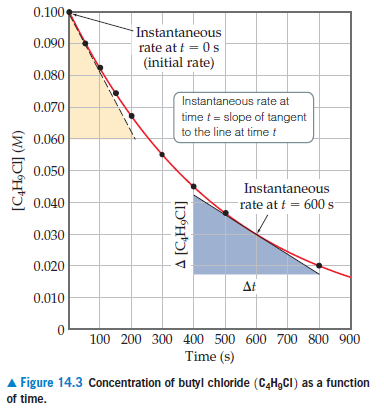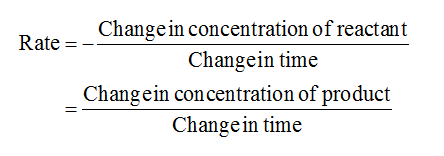# 0.100Instantaneousrate at t = 0 s(initial rate)0.0900.080Instantaneous rate at0.070time t = slope of tangentto the line at time t0.0600.050Instantaneous0.040rate at t = 600 s0.0300.020Δ0.010100 200 300 400 500 600 700 800 900Time (s)A Figure 14.3 Concentration of butyl chloride (C,H,CI) as a functionof time.[C‚H,CI] (M)[1D°H*ɔ] v

Question
1 views

Using Figure 14.3, calculate the instantaneous rate of disappearance of C4H9Cl at t = 0 s (the initial rate).help_outlineImage Transcriptionclose0.100 Instantaneous rate at t = 0 s (initial rate) 0.090 0.080 Instantaneous rate at 0.070 time t = slope of tangent to the line at time t 0.060 0.050 Instantaneous 0.040 rate at t = 600 s 0.030 0.020 Δ 0.010 100 200 300 400 500 600 700 800 900 Time (s) A Figure 14.3 Concentration of butyl chloride (C,H,CI) as a function of time. [C‚H,CI] (M) [1D°H*ɔ] v fullscreen
check_circle

Step 1

The instantaneous rate for the reaction is calculated by the formula given below....

### Want to see the full answer?

See Solution

#### Want to see this answer and more?

Solutions are written by subject experts who are available 24/7. Questions are typically answered within 1 hour.*

See Solution
*Response times may vary by subject and question.
Tagged in